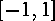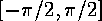<b>double asin(double x)</b>

Document: The C Standard Librarydouble acos(double x)Mathematical Functions: math.hdouble tan(double x)

## double asin(double x)

The return value is the inverse sine or arcsine of x. x must be in the interval. The return value will be expressed in radians and lie in the interval.

McMullin@eeng.dcu.ie
Fri Mar 29 14:35:38 GMT 1996